Module 21 - Answers Lesson 1 Answer 1 21.1.1   The first ten terms of the sequence are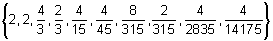As n gets larger subsequent terms in the sequence get closer to zero.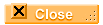Answer 2 21.1.2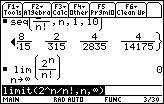The limit of the sequence as n approachesis 0.Answer 3 21.1.3   The points in the graph level off and approach a horizontal line. In other words, the graph has a horizontal asymptote.Answer 4 21.1.4   Plotting the terms of the sequence gives graphical evidence that the sequence converges. The window shown is [xmin, xmax] x [ymin, ymax] = [0, 20] x [2, 3] with nmin = 1 and nmax = 20.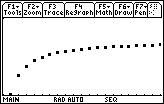A table of values of the terms of the sequence gives numerical evidence that the sequence converges.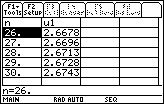The value of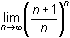gives analytical proof that the sequence converges.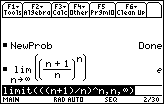The sequence converges to e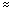2.71828.Lesson 2 Answer 1 21.2.1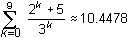Answer 2 21.2.2   The fact that the graph appears to level off and have a horizontal asymptote suggests that the sequence of partial sums may converge, which would mean that the infinite series converges.Answer 3 21.2.3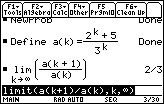Because the limit of the ratio of the sequence of partial sums is less than 1, the series converges.Answer 4 21.2.4   In the previous table the 26th and 51st partial sums were both approximately 10.5. So a reasonable estimate for the sum of the infinite series is 10.5.Lesson 3 Answer 1 21.3.1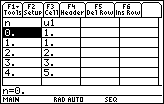The sequence is known as the Fibonacci sequence: 1, 1, 2, 3, 5, 8, 13, 21, 34,...Self Test Answer 1Answer 2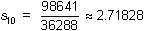Answer 3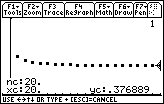The sequence appears to converge to approximately 0.377. The actual value is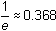Answer 4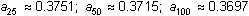Answer 5 Set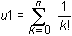in the Y= Editor.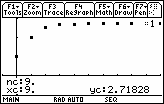The series appears to converge to approximately 2.71828. The exact value is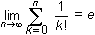Answer 6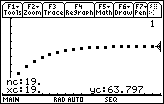The sequence appears to converge to approximately 63.797. The exact value is 64.©Copyright 2007 All rights reserved. | Trademarks | Privacy Policy | Link Policy